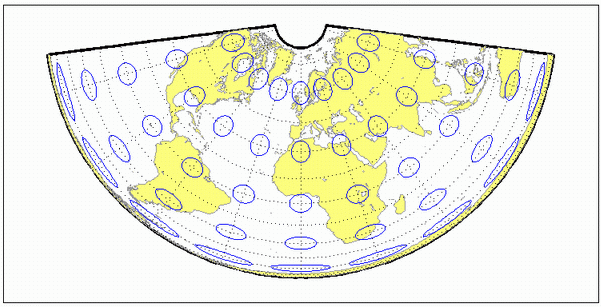Documentation

# eqdconic

Equidistant Conic Projection

Conic

## Identifier

`eqdconic`

## Graticule

Meridians: Equally spaced straight lines converging to a common point, usually beyond the pole. The angles between the meridians are less than the true angles.

Parallels: Equally spaced concentric circular arcs centered on the point of meridional convergence.

Poles: Normally circular arcs, enclosing the same angle as the displayed parallels.

## Features

Scale is true along each meridian and the one or two selected standard parallels. Scale is constant along any parallel. This projection is free of distortion along the two standard parallels. Distortion is constant along any other parallel. This projection provides a compromise in distortion between conformal and equal-area conic projections, of which it is neither.

## Parallels

The cone of projection has interesting limiting forms. If a pole is selected as a single standard parallel, the cone is a plane, and an Equidistant Azimuthal projection results. If two parallels are chosen, not symmetric about the Equator, then an Equidistant Conic projection results. If a pole is selected as one of the standard parallels, then the projected pole is a point, otherwise the projected pole is an arc. If the Equator is so chosen, the cone becomes a cylinder and a Plate Carrée projection results. If two parallels equidistant from the Equator are chosen as the standard parallels, an Equidistant Cylindrical projection results. The default parallels are [15 75].

## Remarks

In a rudimentary form, this projection dates back to Claudius Ptolemy, about A.D. 100. Improvements were developed by Johannes Ruysch in 1508, Gerardus Mercator in the late 16th century, and Nicolas de l'Isle in 1745. It is also known as the Simple Conic or Conic projection.

## Limitations

Longitude data greater than 135º east or west of the central meridian is trimmed.

## Example

```landareas = shaperead('landareas.shp','UseGeoCoords',true); axesm ('eqdconic', 'Frame', 'on', 'Grid', 'on'); geoshow(landareas,'FaceColor',[1 1 .5],'EdgeColor',[.6 .6 .6]); tissot;````eqdconicstd`